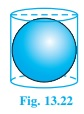# A right circular cylinder just encloses a sphere of radius r (see fig.) . Find (i) surface area of the sphere, (ii) curved surface area of the cylinder, (iii) ratio of the areas obtained in(i) and (ii)(i) Surface area of sphere = 4πr², where r is the radius of sphere

(ii) As Height of cylinder, h = r+r =2r

And Radius of cylinder = r

CSA of cylinder formula = 2πrh = 2πr(2r) (using value of h)

= 4πr²

(iii) Ratio between areas = (Surface area of sphere)/CSA of Cylinder)

= 4r2/4r2 = 1/1

Therefore, Ratio of the areas obtained in (i) and (ii) is 1:1.